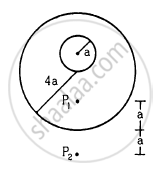Advertisement Remove all ads

# A Uniform Metal Sphere of Radius a and Mass M is Surrounded by a Thin Uniform Spherical Shell of Equal Mass and Radius 4a (Figure 11−E2). - Physics

Sum

A uniform metal sphere of radius a and mass M is surrounded by a thin uniform spherical shell of equal mass and radius 4a (In the following figure). The centre of the shell falls on the surface of the inner sphere. Find the gravitational field at the points P1 and P2 shown in the figure.Advertisement Remove all ads

#### Solution

At point P1, the gravitational field due to the sphere and the shell is given by
F $= \frac{GM}{\left( 3a + a \right)^2} + 0 = \frac{GM}{16 a^2}$

At point P2, the gravitational field due to the sphere and the shell is given by

$F = \frac{GM}{\left( a + 4a + a \right)^2} + \frac{GM}{\left( 4a + a \right)^2}$

$\Rightarrow F = \frac{GM}{36 a^2} + \frac{GM}{25 a^2}$

$\Rightarrow F = \frac{GM}{a^2}\left( \frac{1}{36} + \frac{1}{25} \right)$

$\Rightarrow F = \frac{GM}{a^2}\left( \frac{25 + 36}{900} \right)$

$\Rightarrow F = \left( \frac{61}{900} \right)\frac{GM}{a^2}$

Is there an error in this question or solution?
Advertisement Remove all ads

#### APPEARS IN

HC Verma Class 11, 12 Concepts of Physics 1
Chapter 11 Gravitation
Q 14 | Page 226
Advertisement Remove all ads
Advertisement Remove all ads
Share
Notifications

View all notifications

Forgot password?